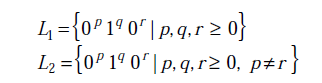# GATE | GATE CS 2013 | Question 32

Consider the following languages.Which one of the following statements is FALSE?
(A) L2 is context-free.
(B) L1 intersection L2 is context-free.
(C) Complement of L2 is recursive.
(D) Complement of L1 is context-free but not regular.

Explanation: (D) is false.

L1 is regular, so its complement would also be regular.

L1 is a regular language of the form 0^* 1^* 0^*. L2 on the other hand is a CFL as it can be derived from the following CFG
L2 = { 0^p 1^q 0^r | p,q,r>0   And p notEqualTo r }
S -> AC|CA
C -> 0C0|B
A -> 0A|0
B -> 1B|epsilon
If coming up with a CFG for L2 is difficult, one can intuitively see that by reducing it to a simpler problem. L2 is very similar to a known CFL L3 = { a^m b^l | m notEqualTo n }

(A) L2 is context free, which is true [CORRECT]
(B) L1 intersection L2 is context free, which is again true because L1 is a regular language and L2 is a CFL. RL union CFL is always a CFL. Hence [CORRECT]
(C) Complement of L2 is recursive, which is true due to the fact that complement of a CFL is CSL for sure (Context sensitive language), which in turn (CSL) is a subset of recursive languages. Hence [CORRECT]
(D) Complement of L1 is context free but not regular, which is false due to closure laws of regular languages. Complement of a RL is always a RL. Hence [INCORRECT]

This solution is contributed by Vineet Purswani .

Quiz of this Question

My Personal Notes arrow_drop_up
Article Tags :

1

Please write to us at contribute@geeksforgeeks.org to report any issue with the above content.